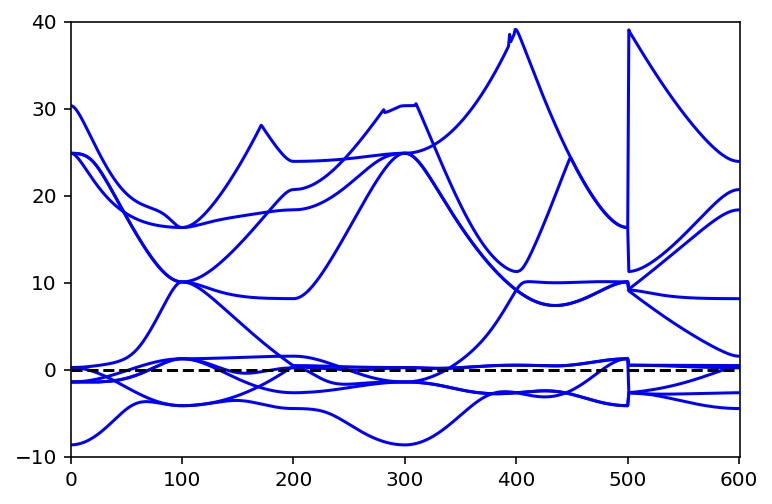# Band structure calculations¶

In this notebook we will see, how to calculate band structures using pyiron.

In :
from pyiron import Project
from ase.spacegroup import crystal
import matplotlib.pyplot as plt
import seekpath as sp
import numpy as np
%config InlineBackend.figure_format = 'retina'

In :
pr = Project("band_structure")


# Structures with seekpath¶

First we see how seekpath works! Therefore we create firts a structure using pyiron.

## Create structure with pyiron¶

In :
structure_Fe = pr.create_structure("Fe", "bcc", 2.81)

In :
structure_Fe.plot3d()

In :
structure_Fe

Out:
Fe: [0. 0. 0.]
Fe: [1.405 1.405 1.405]
pbc: [ True  True  True]
cell:
[[2.81000000e+00 0.00000000e+00 0.00000000e+00]
[1.72062875e-16 2.81000000e+00 0.00000000e+00]
[1.72062875e-16 1.72062875e-16 2.81000000e+00]]

## Create structure with seekpath¶

For seekpath we need a tuple containing

1. The cell in $3\times3$ array
2. The scaled positions
3. List of ints to distinguish the atom types (indices of pyiron structure) as input structure.
In :
input_sp = (structure_Fe.cell, structure_Fe.get_scaled_positions(), structure_Fe.indices)


Just to see how the output looks like, let us do...

In :
sp.get_path(input_sp)

Out:
{'point_coords': {'GAMMA': [0.0, 0.0, 0.0],
'H': [0.5, -0.5, 0.5],
'P': [0.25, 0.25, 0.25],
'N': [0.0, 0.0, 0.5]},
'path': [('GAMMA', 'H'),
('H', 'N'),
('N', 'GAMMA'),
('GAMMA', 'P'),
('P', 'H'),
('P', 'N')],
'has_inversion_symmetry': True,
'augmented_path': False,
'bravais_lattice': 'cI',
'bravais_lattice_extended': 'cI1',
'conv_lattice': array([[2.81, 0.  , 0.  ],
[0.  , 2.81, 0.  ],
[0.  , 0.  , 2.81]]),
'conv_positions': array([[0. , 0. , 0. ],
[0.5, 0.5, 0.5]]),
'conv_types': array([0, 0], dtype=int32),
'primitive_lattice': array([[-1.405,  1.405,  1.405],
[ 1.405, -1.405,  1.405],
[ 1.405,  1.405, -1.405]]),
'primitive_positions': array([[0., 0., 0.]]),
'primitive_types': array(, dtype=int32),
'reciprocal_primitive_lattice': [[1.6776982741209693e-16,
2.236009006113732,
2.236009006113732],
[2.236009006113732, 0.0, 2.236009006113732],
[2.236009006113732, 2.236009006113732, 0.0]],
'inverse_primitive_transformation_matrix': array([[0, 1, 1],
[1, 0, 1],
[1, 1, 0]]),
'primitive_transformation_matrix': array([[-0.5,  0.5,  0.5],
[ 0.5, -0.5,  0.5],
[ 0.5,  0.5, -0.5]]),
'volume_original_wrt_conv': 0.9999999999999999,
'volume_original_wrt_prim': 1.9999999999999998,
'spacegroup_number': 229,
'spacegroup_international': 'Im-3m'}

The code creates automatically the conventional and primitive cell with all high-symmetry points and a suggested path taking all high-symmetry paths into account.

Keep in mind: The high-symmetry points and paths make only sence for the primitive cell! Therefore we run all calculations in the primitive cell created by seekpath.

## Create a structure¶

We use the same structure as before!

## Create new structure (primitive cell) with high-symmetry points and paths¶

For the following command all arguments valid for seekpath are supported. Look at the docstring and at seekpath.

In :
structure_Fe_sp = structure_Fe.create_line_mode_structure()

In :
structure_Fe_sp.plot3d()

In :
structure_Fe_sp

Out:
Fe: [0. 0. 0.]
pbc: [ True  True  True]
cell:
[[-1.405  1.405  1.405]
[ 1.405 -1.405  1.405]
[ 1.405  1.405 -1.405]]

We see, that the structure is now the primitive cell containing only one atom.

In :
structure_Fe_sp.get_high_symmetry_points()

Out:
{'GAMMA': [0.0, 0.0, 0.0],
'H': [0.5, -0.5, 0.5],
'P': [0.25, 0.25, 0.25],
'N': [0.0, 0.0, 0.5]}
In :
structure_Fe_sp.get_high_symmetry_path()

Out:
{'full': [('GAMMA', 'H'),
('H', 'N'),
('N', 'GAMMA'),
('GAMMA', 'P'),
('P', 'H'),
('P', 'N')]}

The path is stored like this. Here you can also add paths to the dictionary.

Each tuple gives a start and end point for this specific trace. Thus also disonnected paths are possible to calculate.

In :
structure_Fe_sp.add_high_symmetry_path({"my_path": [("GAMMA", "H"), ("GAMMA", "P")]})

In :
structure_Fe_sp.get_high_symmetry_path()

Out:
{'full': [('GAMMA', 'H'),
('H', 'N'),
('N', 'GAMMA'),
('GAMMA', 'P'),
('P', 'H'),
('P', 'N')],
'my_path': [('GAMMA', 'H'), ('GAMMA', 'P')]}

## Create jobs¶

We need two jobs for a band structure! The first gives us the correct Fermi energy and the charge densities used for the second calculations.

## Create job for charge density¶

This is only a small example for BS calculations. Could be that the input parameter like cutoff etc. does not make much sense... for real physics...

In :
def setup_hamiltonian_sphinx(project, jobname, structure, chgcar_file=""):

#version 1.0 (08.03.2019)

#Name und typ
ham = project.create_job(job_type='Sphinx', job_name=jobname)

#parameter für xc functional
ham.exchange_correlation_functional = 'PBE'

#struktur
ham.structure = structure
ham.load_default_groups()

ham.set_encut(450)
ham.set_empty_states(6)
ham.set_convergence_precision(electronic_energy=1e-8)
ham.set_occupancy_smearing(width=0.2)

#parameter für kpoints
ham.set_kpoints([8, 8, 8])

return ham

In :
ham_spx_chg = setup_hamiltonian_sphinx(pr, "Fe_spx_CHG", structure_Fe_sp)


### Run it!¶

In :
ham_spx_chg.run()

The job Fe_spx_CHG was saved and received the ID: 4

In :
pr.get_jobs_status()

Out:
finished    1
Name: status, dtype: int64

## Create second job¶

We restart the fist job with the following command. Then the charge density of the first job is taken for the second!

In :
ham_spx_bs = ham_spx_chg.restart_for_band_structure_calculations(job_name="Fe_spx_BS")

ham_spx_bs = ham_spx_chg.restart_from_charge_density( job_name="Fe_spx_BS", job_type=None, band_structure_calc=True )

### Set line mode for k-points¶

To set the correct path, we have to give the name of the path (in our example either full or my_path) and the number of points for each subpath (would be for n_path=100and path_name="my_path" 200 k-points in total)

In :
ham_spx_bs.set_kpoints(scheme="Line", path_name="full", n_path=100)

/srv/conda/envs/notebook/lib/python3.7/site-packages/pyiron/base/generic/parameters.py:285: UserWarning: The input in GenericParameters changed, while the state of the job was already finished.
"The input in GenericParameters changed, while the state of the job was already finished."


A parameter usefull for BS calculations. Look at the sphinx manual for details.

In :
ham_spx_bs.input["nSloppy"] = 6


### Run it!¶

In :
ham_spx_bs.run()

The job Fe_spx_BS was saved and received the ID: 5

In :
pr.get_jobs_status()

Out:
finished    2
Name: status, dtype: int64

## Store the data!¶

The energy values are stored in the following paths of the hdf5 file.

In :
energy_sphinx = ham_spx_bs['output/generic/dft/bands_eigen_values'][-1]
ef_sphinx = ham_spx_chg['output/generic/dft/bands_e_fermi'][-1]
energy_sphinx -= ef_sphinx


## Plot it!¶

Now we can easily plot it!

In :
plt.plot(energy_sphinx[:-1], 'b-')
plt.axhline(y=0, ls='--', c='k')
plt.xlim(0, len(energy_sphinx));
plt.ylim(-10,40);In [ ]: